# Excel AVERAGEIFS function with multiple criteria

This tutorial shows how to use the Excel AVERAGEIFS function for calculating an average with multiple conditions.

When it comes to calculating an arithmetic mean of a group of numbers in Excel, AVERAGE is the way to go. To average cells that meet a certain condition, AVERAGEIF comes in handy. To find an average with multiple criteria, AVERAGEIFS is the function to use. To learn how it works, please keep reading!

## AVERAGEIFS function in Excel

The Excel AVERAGEIFS function calculates the arithmetic mean of all cells in a range that meet the specified criteria.

The syntax is as follows:

AVERAGEIFS(average_range, criteria_range1, criteria1, [criteria_range2, criteria2], …)

Where:

• Average_range - the range of cells to average.
• Criteria_range1, criteria_range2, … - ranges to be tested against the corresponding criteria.
• Criteria1, criteria2, … - criteria that determine which cells to average. The criteria can be supplied in the form of a number, logical expression, text value, or cell reference.

Criteria_range1 / criteria1 are required, subsequent ones are optional. 1 to 127 range/criteria pairs can be used in one formula.

The AVERAGEIFS function is available in Excel 2007 - Excel 365.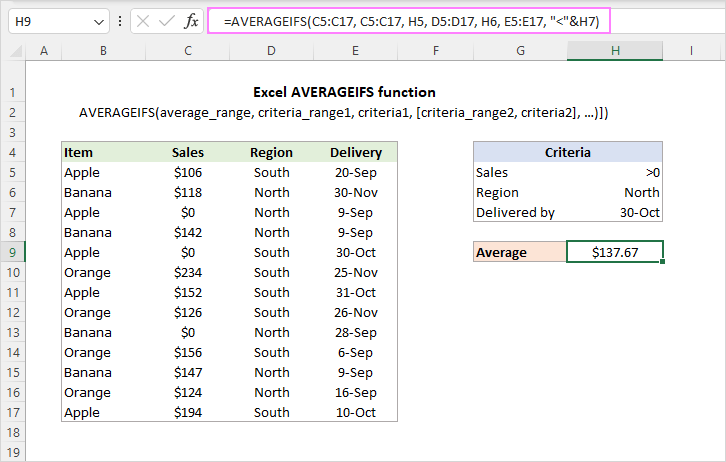Note. The AVERAGEIFS function works with the AND logic, i.e. only those cells are averaged for which all the conditions are TRUE. To calculate cells for which any single condition is TRUE, use the AVERAGE IF OR formula.

### AVERAGEIFS function - usage notes

To get a clear understanding of how the function works and avoid errors, take notice of the following facts:

• In the average_range argument, empty cells, logical values TRUE/FALSE, and text values are ignored. Zero values are included.
• If criteria is an empty cell, it is treated as a zero value.
• If average_range doesn't contain a single numeric value, a #DIV/0! error occurs.
• If no cells meet all of the specified criteria, a #DIV/0! error is returned.
• AVERAGEIFS' criteria may apply to the same range or different ranges.
• Each criteria_range must be of the same size and shape as average_range, otherwise a #VALUE! error occurs.

Now that you know the theory, let's see how to use the AVERAGEIFS function in practice.

## Excel AVERAGEIFS formula

First, let us outline the generic approach. To construct an AVERAGEIFS formula correctly, please follow these guidelines:

1. In the first argument, supply the range that you want to average.
2. In subsequent arguments, specify range/criteria pairs. The pairs can be arranged in any order, but the criteria always follows the range it applies to.
3. An AVERAGEIFS formula should always contain an odd number of arguments: average_range + one or more criteria_range/criteria pairs.

## AVERAGEIFS with text criteria

To get an average of numbers in one column if another column(s) contains certain text, use that text for criteria.

As an example, let's find an average of the "Apple" sales in the "North" region. For this, we make an AVERAGEIFS formula with two criteria:

• Average_range is C3:C15 (cells to average).
• Criteria_range1 is A3:A15 (Items to check) and criteria1 is "apple".
• Criteria_range2 is B3:B15 (Regions to check) and criteria2 is "north".

Putting the arguments together, we get the following formula:

`=AVERAGEIFS(C3:C15, A3:A15, "apple", B3:B15, "north")`

With criteria in predefined cells (F3 and F4), the formula takes this form:

`=AVERAGEIFS(C3:C15, A3:A15, F3, B3:B15, F4)`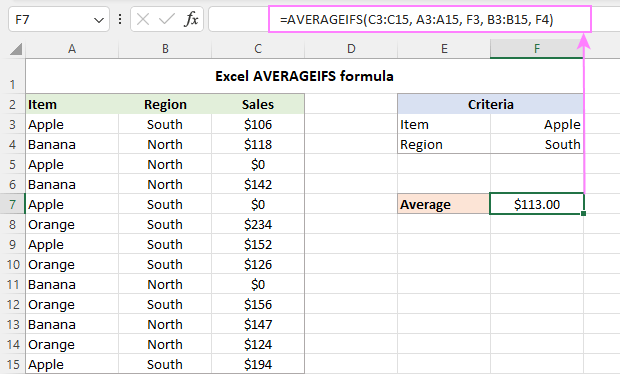## AVERAGEIFS with logical operators

When the criteria default to "is equal to", the equality sign can be omitted, and you simply put the target text (enclosed in quotation marks) or number (without the quotation marks) in the corresponding argument like shown in the previous example.

When using other logical operators such as "greater than" (>), "less than" (<), not equal to (<>), and others with a number or date, you enclose the whole construction in double quotes.

For example, to average sales greater than zero delivered by 1-Oct-2022, the formula is:

`=AVERAGEIFS(C3:C15, B3:B15, "<10/1/2022", C3:C15, ">0")`

When the criteria are in separate cells, you enclose a logical operator in quotation marks and concatenate it with a cell reference using an ampersand (&). For example:

`=AVERAGEIFS(C3:C15, B3:B15, "<"&F3, C3:C15, ">"&F4)`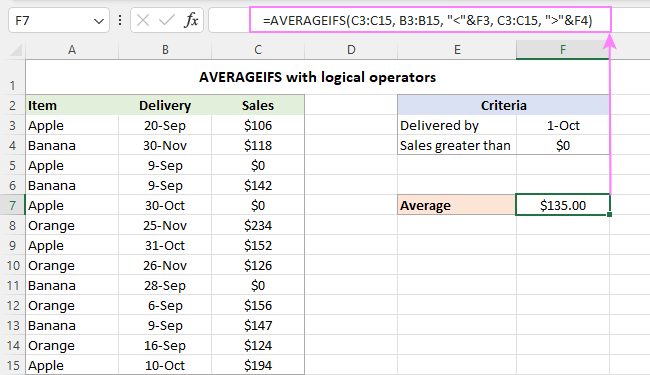## AVERAGEIFS with wildcard characters

To average cells based on partial text match, use wildcard characters in criteria - a question mark (?) to match any single character or an asterisk (*) to match any number of characters.

In the table below, suppose you wish to average "orange" sales in all "south" regions including "south-west" and "south-east". To have it done, we include an asterisk in the second criteria:

`=AVERAGEIFS(C3:C15, A3:A15, F3, B3:B15, "south*")`

If a partial text match criteria is input in a cell, then concatenate a wildcard character with the cell reference. In our case, the formula takes this shape:

`=AVERAGEIFS(C3:C15, A3:A15, F3, B3:B15, F4&"*")`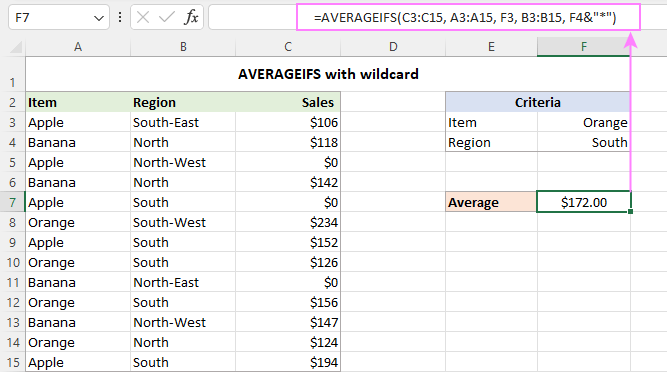## Average if between two values

To get the average of values that fall between two specific values, use one of the following generic formulas:

Average if between two values, inclusive:

AVERAGEIFS(average_range, criteria_range,">=value1", criteria_range,"<=value2")

Average if between two values, exclusive:

AVERAGEIFS(average_range, criteria_range,">value1", criteria_range,"<value2")

In the 1st formula, you use the greater than or equal to (>=) and less than or equal to (<=) logical operators, so the boundary values are included in the average.

In the 2nd formula, the greater than (>) and less than (<) logical criteria exclude the boundary values from the average.

These formulas work nicely or both scenarios - when the cells to average and the cells to check are in the same column or in two different columns.

For example, to calculate the average of sales between 100 and 130 inclusive, you can use this formula:

`=AVERAGEIFS(C3:C15, C3:C15, ">=100", C3:C15, "<=130")`

With the boundary values in cells E3 and F3, the formula takes this form:

`=AVERAGEIFS(C3:C15, C3:C15, ">="&E3, C3:C15, "<="&F3)`

Please notice that in this case we use the same reference (C3:C15) for the 3 range arguments.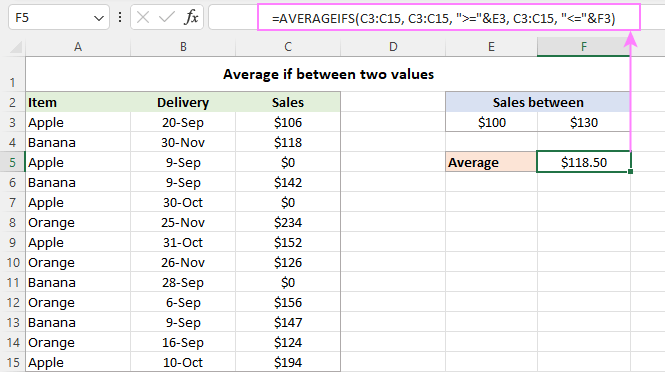To average cells in a given column if the values in another column fall between two values, supply a different range for the average_range and criteria_range arguments.

For instance, to average the sales in column C if the date in column B is between 1-Sep and 30-Oct, the formula is:

`=AVERAGEIFS(C3:C15, B3:B15, ">=9/1/2022", B3:B15, "<=10/30/2022")`

With cell references:

`=AVERAGEIFS(C3:C15, B3:B15, ">="&E3, B3:B15, "<="&F3)`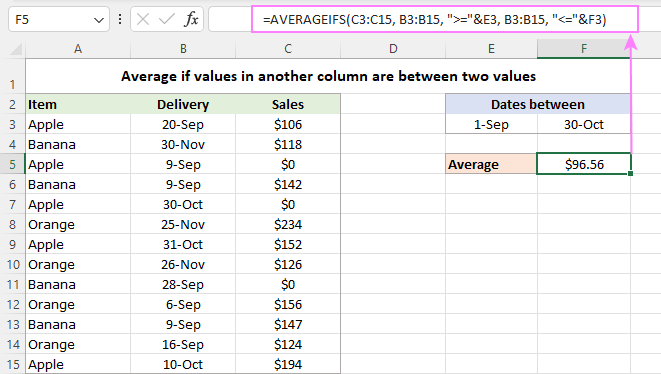That's how you use the AVERAGEIFS function in Excel to find an arithmetic mean with multiple criteria. I thank you for reading and hope to see you on our blog next week!

Excel AVERAGEIFS function - examples (.xlsx file)

## You may also be interested in

1. Hi! I am trying to have many arguments for the averageif but all in the same column
example: for the data below, I want to get an average of the values in column 3 if column 2 is "red" "pink" or "blue". how would i do this?

col 1 // col 2 // col 3
A / blue // 2.5
B // red // 3
A / pink// 2.5
A // grey// 3
c / red// 2.5
B // pink// 3

• Hi! Please re-check the article above since it covers your case. For example,

=AVERAGEIFS(C1:C10,B1:B10,"red")

• Since AVERAGEIFS only lets you look for 1 criteria per column, you'll need to set up a helper column. i.e.,

=OR(COUNTIF(B3,"red"),COUNTIF(B3,"pink"),COUNTIF(B3,"blue"))

Then make the criteria range the helper column you set up and have your criteria be "TRUE"

2. Thank you for your quick reply! In this example, that is indeed a good solution. However, some cities do not have that Youth Movement (1). In that case, I need to refer to another Youth movement, but if that movement is also active in one or more of the other cities, it will duplicate the amount of locations and take it into the calculation. I'm sorry if I'm explaining it a bit unclear.

3. I see that the formatting of my example is staggered unfortunately. Therefore I try it again.
Note: City = Column A, Youth Movement = Column B, Amount of locations = column C.

City (A) - Youth Movement (B) - Amount of locations (C)
Row 1 New York - Youth Movement 1 - 5
Row 2 New York - Youth Movement 3 - 5
Row 3 New York - Youth Movement 5 - 5
Row 4 Sydney - Youth Movement 1 - 3
Row 5 Sydney - Youth Movement 3 - 3
Row 6 Sydney - Youth Movement 4 - 3
Row 7 Sydney - Youth Movement 6 - 3

4. Hi,

Thanks for all the tutorials. They are of a great help for me.
However, I've got a problem and I can't figure out how to solve it. I want to calculate the average amount of locations of youth movements of several cities, but the problem is that the number of locations given in the collumn is of all the movements, while in the collumn of the youth movements distinguishes between the type of movements, and not every city has the same sort and amount of movements. The result is that the average that I calculate is not the average of the locations in the cities. Here's an example to illustrate my issue:

(A) Place (B) Youth movement (C) Amount of locations
1. New York YM1 5
2. New York YM3 5
3. New York YM5 5
4. Sydney YM1 3
5. Sydney YM3 3
6. Sydney YM 4 3
7. Sydney YM 6 3

So, while the average should be 4, based on my formula, I get 3.86 as a result, because it gives the average of the sum of all locations. Because it is a pretty big datasets with lots of city combinations, it’s almost impossible to calculate it manually. Does anyone has an idea?